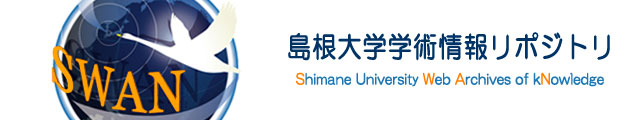ダウンロード数 : ?
 言語 英語 著者 Ishihara, Kazuki Department of Mathematics, Shimane University 内容記述（抄録等） The present paper deals with the problem of oscillation for a second-order linear differential equation in which an impulsive effect is considered. This equation can be regarded as an equation of motion in which the moving speed of a mass point changes sharply by some influence. It is proved that there is a case that the mass point may oscillate due to the influence of the impulsive effect even if the mass point does not oscillate in the model removing the impulsive effect. It is also shown that the obtained results extend some previous ones through the use of an example. 主題 Oscillation problem Impulse Riccati transformation Integral averaging technique 掲載誌名 Journal of Mathematical Analysis and Applications 巻 470 号 2 開始ページ 911 終了ページ 930 ISSN 0022-247X 発行日 2019-02 DOI 10.1016/j.jmaa.2018.10.041 出版者 Elsevier 資料タイプ 学術雑誌論文 ファイル形式 PDF 著者版/出版社版 著者版 部局 総合理工学部